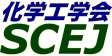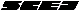## \$B9V1i%W%m%0%i%`!J2q>l!&F|DxJL!K(B

### F\$B2q>l(B \$BBh(B2\$BF|(B

\$B:G=*99?7F|;~!'(B2012-08-20 15:02:17
\$B9V1i(B
\$B;~9o(B
\$B9V1i(B
\$BHV9f(B
\$B9V1iBjL\!?H/I=\$B%-!<%o!<%I(B\$BJ,N`(B
\$BHV9f(B
\$B\$BHV9f(B
\$B%7%s%]%8%&%`(B \$B!c:`NA!&3&LLF\$O@2q!V:`NAAO@.\$H3&LL8=>]!W!d(B
(9:00\$B!A(B10:00)\$B!!(B(\$B:BD9(B \$BEOn5(B \$BE/(B)
9:00\$B!A(B 9:20F201\$B%U%)%H%K%C%/%P%s%H\$H%W%i%:%b%K%/%9\$N%O%\$%V%j%C%I8z2L\$r5/\$3\$9%J%N9=B\$\$N:n@=(B
(\$BFAEgBg1!%=%7%*%F%/%N%5%\$%(%s%9(B) \$B!{(B(\$B@5(B)\$B?9(B \$BFF;K(B \$B!&(B (\$BFAEgBg1!@hC<2J3X5;=Q(B) \$BEl(B \$B?-8g(B \$B!&(B (\$BFAEgBg1!%=%7%*%F%/%N%5%\$%(%s%9(B) (\$B@5(B)\$BNkLZ(B \$BNI>0(B \$B!&(B (\$BL>;TBg1!Lt(B) \$BK-6L(B \$B>4;R(B \$B!&(B \$B;3Cf(B \$B=_J?(B \$B!&(B (\$BJ*:`5!9=(B) \$B_7ED(B \$BJY(B \$B!&(B (\$BFAEgBg1!%=%7%*%F%/%N%5%\$%(%s%9(B) \$B66K\(B \$B=\$0l(B \$B!&(B \$B868}(B \$B2m@k(B
gel-immobilized colloidal crystal
photonic crystal
plasmonics
S-29621
9:20\$B!A(B 9:40F202\$B:Y9&3+JD5!G=\$rM-\$9\$k%\$%*%s8r49BN\$N3+H/(B
(\$B=)EDBg1!9);q(B) \$B!{(B(\$B@5(B)\$BB<>e(B \$B8-<#(B \$B!&(B (\$B@5(B)\$B2CF#(B \$B5.9((B \$B!&(B (\$B@5(B)\$B?{86(B \$B>!9/(B
Mesoporous silica
Thermoresponsive polymer
Ion exchange
S-29448
9:40\$B!A(B 10:00F203\$B?e>t2=\$K\$*\$1\$k(BTiO2\$B8w?(G^\$N3+H/(B
(\$B%Q%J%=%K%C%/@hC<8&(B) \$B!{(B(\$B@5(B)\$B4]Hx(B \$B\$f\$&\$3(B \$B!&(B \$BCvLn(B \$BBgJe(B \$B!&(B (\$B@5(B)\$B9b2,(B \$BM'9/(B \$B!&(B \$B9TE7(B \$B5WO/(B
titanium dioxide
water purification
photocatalyst
S-29379
(10:00\$B!A(B11:00)\$B!!(B(\$B:BD9(B \$BB<>e(B \$B8-<#(B)
10:00\$B!A(B 10:20F204*BEA\$B7?%8%s%3%"%k%_%N%7%j%1!<%H%<%*%i%\$%H\$NM-5!9=B\$5,Dj:^L5E:2C9g@.\$H5[CeFC@-(B
(\$BElBg1!9)(B) \$B!{(B(\$B3X(B)\$B0KgPLZ(B \$B7rB@(B \$B!&(B \$BHD66(B \$B7D<#(B \$B!&(B (\$B@5(B)\$BBg5WJ](B \$BC#Li(B
zeolite beta
zincoaluminosilicate
seed-assisted synthesis
S-29410
10:20\$B!A(B 10:40F205\$B%\$%s%?!<%G%8%F!<%H7?B?9&@-G[0L9bJ,;R\$,<(\$95[CeM65/9=B\$E>0\8=>]\$N%b%G%k2=(B
(\$B5~Bg9)(B) \$B!{(B(\$B3X(B)\$B>B8}(B \$BNKJ?(B \$B!&(B \$B;3K\(B \$B0&(B \$B!&(B (\$B@5(B)\$B?y;3(B \$BH;?M(B \$B!&(B (\$B@5(B)\$BEDCf(B \$B=( \$B!&(B (\$B@5(B)\$BEOn5(B \$BE/(B \$B!&(B \$BJ!Eg(B \$BCN9((B \$B!&(B \$BKYLS(B \$B8g;K(B \$B!&(B \$BKL@n(B \$B?J(B \$B!&(B (\$B@5(B)\$B5\86(B \$BL-(B
Porous Coordination Polymer
Metal-Organic Framework
S-29272
10:40\$B!A(B 11:00F206\$B%J%N:Y9&FbNW3&E@\$NJ,;R%7%_%e%l!<%7%g%s\$K\$h\$kFCDj\$H\$=\$N9M;!(B
(\$B5~Bg9)(B) \$B!{(B(\$B@5(B)\$B5\86(B \$BL-(B \$B!&(B (\$B3X(B)\$BJ!2,(B \$B63J<(B \$B!&(B (\$B@5(B)\$BEDCf(B \$B=(
critical behavior of confined fluids
molecular simulation
S-2947
(11:00\$B!A(B12:00)\$B!!(B(\$B:BD9(B \$B<58M(B \$B>;9-(B)
(\$B5~Bg9)(B) \$B!{(B(\$B3X(B)\$B;0Ln(B \$BBY;V(B \$B!&(B (\$B@5(B)\$BEOn5(B \$BE/(B \$B!&(B (\$B@5(B)\$B5\86(B \$BL-(B
Colloidal patterning
Convective self-assembly
Direct observation
S-29409
11:20\$B!A(B 11:40F208\$B%3%m%\$%IN3;R\$N?eJ?0\N.=8@Q\$K\$h\$kG[NsGvKl7A@.
(\$B5~Bg9)(B) \$B!{(B(\$B@5(B)\$BEOn5(B \$BE/(B \$B!&(B (\$B3X(B)\$BNkLZ(B \$B=_Li(B \$B!&(B (\$B3X(B)\$B;3:,(B \$BM\$0l(B \$B!&(B (\$B7*K\oD9)=j(B) \$B?"ED(B \$B2m8J(B \$B!&(B \$BJ!0f(B \$BIp5W(B \$B!&(B (\$B5~Bg9)(B) (\$B@5(B)\$B5\86(B \$BL-(B
Convective self-assembly
Monolayer
Colloid
S-29992
11:40\$B!A(B 12:00F209\$BHyN3;RJ,;61U\$N4pHD>e4%Ag\$K\$h\$kN3;R7OLVL\9=B\$\$N7A@.%7%_%e%l!<%7%g%s(B
(\$B=;M'Bg:e%;%a%s%H(B) \$B!{(B(\$B@5(B)\$B \$B!&(B (\$BElBg1!9)(B) (\$B@5(B)\$BF#ED(B \$B>;Bg(B \$B!&(B (\$B@5(B)\$B>.CS(B \$B=\$(B \$B!&(B (\$B@5(B)\$B;38}(B \$BM34tIW(B
suspension
network structure
drying simulation
S-29649

\$B9V1i%W%m%0%i%`(B
\$B2=3X9)3X2q(B \$BBh(B44\$B2s=)5(Bg2q(B(C) 2012 \$B8x1W
Most recent update: 2012-08-20 15:02:17
For more information contact \$B2=3X9)3X2qElKL;YIt(B \$BBh(B44\$B2s=)5(Bg2q(B \$BLd\$\$9g\$;78(B
E-mail: inquiry-44fwww3.scej.org
This page was generated byeasp 2.28; proghtml 2.28c (C)1999-2011 kawase